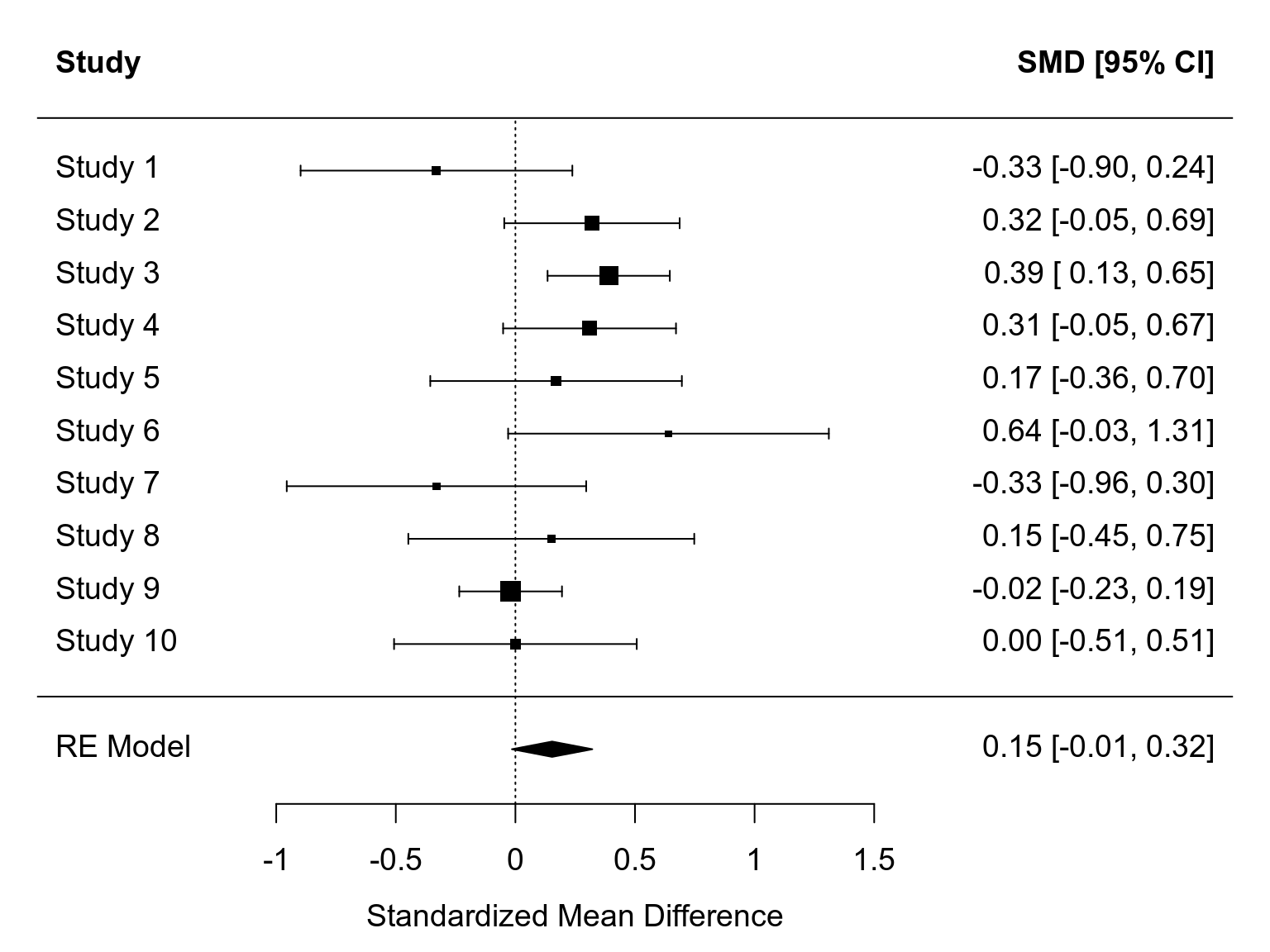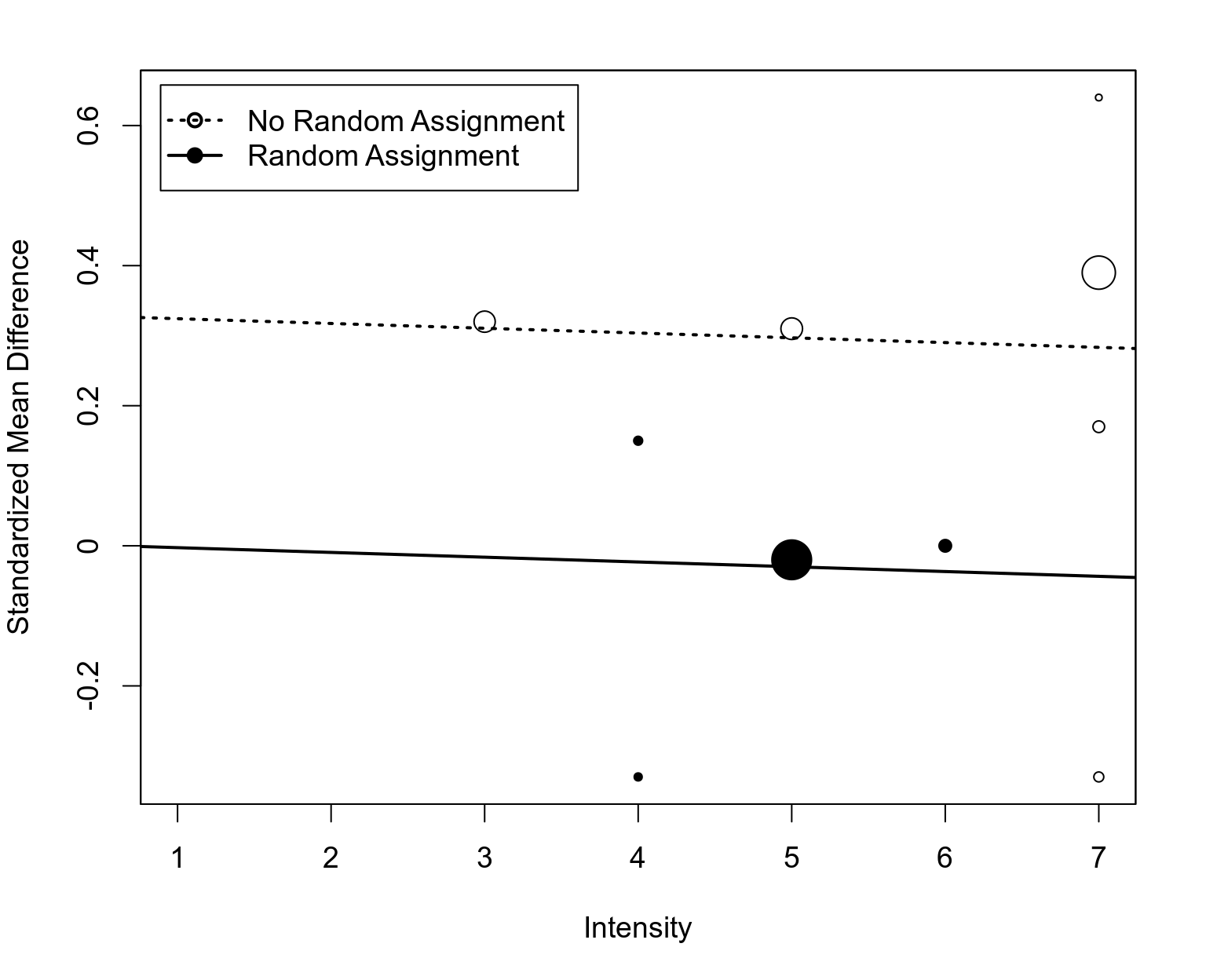## General Notes / Setup

The book Practical Meta-Analysis by Lipsey and Wilson (2001) is an excellent introduction to meta-analysis and covers (in less than 250 pages) the most important aspects of conducting a meta-analysis. This document provides the R code to reproduce the analyses conducted in chapter 7 (which is focused on the computational aspects of a meta-analysis, including the equal-, random-, and the mixed-effects model) using the metafor package. To read more about the package, see the package website and the package documentation.

The package can be installed with:

install.packages("metafor")

Once the package is installed, we can load it with:

library(metafor)

## 1) Data

The data used for the analyses are provided in the book on page 130 in Table 7.1. We can create the same dataset with:

dat <- data.frame(
id = c(100, 308, 1596, 2479, 9021, 9028, 161, 172, 537, 7049),
yi = c(-0.33, 0.32, 0.39, 0.31, 0.17, 0.64, -0.33, 0.15, -0.02, 0.00),
vi = c(0.084, 0.035, 0.017, 0.034, 0.072, 0.117, 0.102, 0.093, 0.012, 0.067),
random = c(0, 0, 0, 0, 0, 0, 1, 1, 1, 1),
intensity = c(7, 3, 7, 5, 7, 7, 4, 4, 5, 6))
dat
##      id    yi    vi random intensity
## 1   100 -0.33 0.084      0         7
## 2   308  0.32 0.035      0         3
## 3  1596  0.39 0.017      0         7
## 4  2479  0.31 0.034      0         5
## 5  9021  0.17 0.072      0         7
## 6  9028  0.64 0.117      0         7
## 7   161 -0.33 0.102      1         4
## 8   172  0.15 0.093      1         4
## 9   537 -0.02 0.012      1         5
## 10 7049  0.00 0.067      1         6


The yi values are standardized mean differences based on studies examining the effectiveness of ‘challenge programs’ to treat juvenile delinquency (presumably with positive values indicating that delinquent behaviors were reduced in the group of juveniles participating in such a challenge program compared to those who did not). The vi values are the corresponding sampling variances. The dummy variable random indicates whether juveniles were randomly assigned to the two conditions with a study (1 = yes, 0 = no). Finally, intensity is a variable coded for the purposes of the meta-analysis which indicates (based on assessment of the coder) the intensity of the challenge program (coded from 1 = ‘very low’ to 7 = ‘very high’). These last two variables are potential moderators of the treatment effectiveness.

## 2) Equal-Effects Model

We can fit an equal-effects model to these data with:

res.ee <- rma(yi, vi, data=dat, method="EE")
res.ee
## Equal-Effects Model (k = 10)
##
## I^2 (total heterogeneity / total variability):   39.04%
## H^2 (total variability / sampling variability):  1.64
##
## Test for Heterogeneity:
## Q(df = 9) = 14.7640, p-val = 0.0976
##
## Model Results:
##
## estimate      se    zval    pval   ci.lb   ci.ub
##   0.1549  0.0609  2.5450  0.0109  0.0356  0.2742  *
##
## ---
## Signif. codes:  0 '***' 0.001 '**' 0.01 '*' 0.05 '.' 0.1 ' ' 1


These results match what is reported on page 132-133 (see Exhibit 7.3).

## 3) Random-Effects Model

A random-effects model (using the DerSimonian-Laird estimator for $$\tau^2$$) can be fitted with:

res.re <- rma(yi, vi, data=dat, method="DL")
res.re
## Random-Effects Model (k = 10; tau^2 estimator: DL)
##
## tau^2 (estimated amount of total heterogeneity): 0.0260 (SE = 0.0328)
## tau (square root of estimated tau^2 value):      0.1611
## I^2 (total heterogeneity / total variability):   39.04%
## H^2 (total variability / sampling variability):  1.64
##
## Test for Heterogeneity:
## Q(df = 9) = 14.7640, p-val = 0.0976
##
## Model Results:
##
## estimate      se    zval    pval    ci.lb   ci.ub
##   0.1534  0.0858  1.7893  0.0736  -0.0146  0.3215  .
##
## ---
## Signif. codes:  0 '***' 0.001 '**' 0.01 '*' 0.05 '.' 0.1 ' ' 1


Again, these results match what is shown in Exhibit 7.3 and on pages 134-135.

A forest plot showing the results of the individual studies and the results from the random-effects model can be created as follows:

par(mar=c(4,4,2,2))

forest(res.re, xlim=c(-2,3), header=c("Study", "SMD [95% CI]"), top=2,
xlab="Standardized Mean Difference")## 4) Analog to the ANOVA

Next, we can carry out the ANOVA-type analysis described on pages 135-138. In particular, a fixed-effects meta-regression model with the random variable as moderator can be fitted with:

res.anova <- rma(yi, vi, mods = ~ random, data=dat, method="FE")
res.anova
## Fixed-Effects with Moderators Model (k = 10)
##
## I^2 (residual heterogeneity / unaccounted variability): 0.00%
## H^2 (unaccounted variability / sampling variability):   0.96
## R^2 (amount of heterogeneity accounted for):            41.40%
##
## Test for Residual Heterogeneity:
## QE(df = 8) = 7.6901, p-val = 0.4643
##
## Test of Moderators (coefficient 2):
## QM(df = 1) = 7.0739, p-val = 0.0078
##
## Model Results:
##
##          estimate      se     zval    pval    ci.lb    ci.ub
## intrcpt    0.2984  0.0813   3.6687  0.0002   0.1390   0.4578  ***
## random    -0.3261  0.1226  -2.6597  0.0078  -0.5664  -0.0858   **
##
## ---
## Signif. codes:  0 '***' 0.001 '**' 0.01 '*' 0.05 '.' 0.1 ' ' 1


Note that the $$Q_E$$ and $$Q_M$$ statistics add up to the $$Q$$ statistic given earlier for the equal-effects models (i.e., $$7.6901 + 7.0739 = 14.7640$$).

The tests for heterogeneity within the two subgroups formed by the moderator can be obtained with:

res.r0 <- rma(yi, vi, data=dat, method="EE", subset=random==0)
res.r0
## Equal-Effects Model (k = 6)
##
## I^2 (total heterogeneity / total variability):   22.34%
## H^2 (total variability / sampling variability):  1.29
##
## Test for Heterogeneity:
## Q(df = 5) = 6.4382, p-val = 0.2659
##
## Model Results:
##
## estimate      se    zval    pval   ci.lb   ci.ub
##   0.2984  0.0813  3.6687  0.0002  0.1390  0.4578  ***
##
## ---
## Signif. codes:  0 '***' 0.001 '**' 0.01 '*' 0.05 '.' 0.1 ' ' 1

res.r1 <- rma(yi, vi, data=dat, method="EE", subset=random==1)
res.r1
## Equal-Effects Model (k = 4)
##
## I^2 (total heterogeneity / total variability):   0.00%
## H^2 (total variability / sampling variability):  0.42
##
## Test for Heterogeneity:
## Q(df = 3) = 1.2519, p-val = 0.7406
##
## Model Results:
##
## estimate      se     zval    pval    ci.lb   ci.ub
##  -0.0277  0.0917  -0.3017  0.7628  -0.2075  0.1521
##
## ---
## Signif. codes:  0 '***' 0.001 '**' 0.01 '*' 0.05 '.' 0.1 ' ' 1


Here, we note that the two separate $$Q$$ statistics add up to the $$Q_E$$ statistic given earlier (i.e., $$6.4382 + 1.2519 = 7.6901$$).

Finally, the predicted/estimated effect for ‘random = 0’ and ‘random = 1’ can be obtained with:

predict(res.anova, newmods=c(0,1))
##      pred     se   ci.lb  ci.ub
## 1  0.2984 0.0813  0.1390 0.4578
## 2 -0.0277 0.0917 -0.2075 0.1521


All of the values shown in Exhibit 7.4 have now been computed.

## 5) Weighted Regression Analysis

The ‘weighted regression analysis’ described on pages 138-140 is again nothing else than a fixed-effects meta-regression model with moderators. Here, the authors are including both the random and the intensity moderators in the model. This can be done with:

res.wr <- rma(yi, vi, mods = ~ random + intensity, data=dat, method="FE")
res.wr
## Fixed-Effects with Moderators Model (k = 10)
##
## I^2 (residual heterogeneity / unaccounted variability): 8.89%
## H^2 (unaccounted variability / sampling variability):   1.10
## R^2 (amount of heterogeneity accounted for):            33.09%
##
## Test for Residual Heterogeneity:
## QE(df = 7) = 7.6832, p-val = 0.3614
##
## Test of Moderators (coefficients 2:3):
## QM(df = 2) = 7.0807, p-val = 0.0290
##
## Model Results:
##
##            estimate      se     zval    pval    ci.lb    ci.ub
## intrcpt      0.3223  0.2998   1.0752  0.2823  -0.2652   0.9099
## random      -0.3298  0.1304  -2.5286  0.0115  -0.5854  -0.0742  *
## intensity   -0.0041  0.0493  -0.0829  0.9339  -0.1007   0.0925
##
## ---
## Signif. codes:  0 '***' 0.001 '**' 0.01 '*' 0.05 '.' 0.1 ' ' 1


Exhibit 7.6 (page 141) shows the same results.

## 6) Mixed-Effects Model

Finally, the mixed-effects meta-regression model is described on pages 140-142. These results can be obtained with:

res.me <- rma(yi, vi, mods = ~ random + intensity, data=dat, method="DL")
res.me
## Mixed-Effects Model (k = 10; tau^2 estimator: DL)
##
## tau^2 (estimated amount of residual heterogeneity):     0.0049 (SE = 0.0294)
## tau (square root of estimated tau^2 value):             0.0698
## I^2 (residual heterogeneity / unaccounted variability): 8.89%
## H^2 (unaccounted variability / sampling variability):   1.10
## R^2 (amount of heterogeneity accounted for):            81.20%
##
## Test for Residual Heterogeneity:
## QE(df = 7) = 7.6832, p-val = 0.3614
##
## Test of Moderators (coefficients 2:3):
## QM(df = 2) = 5.5711, p-val = 0.0617
##
## Model Results:
##
##            estimate      se     zval    pval    ci.lb    ci.ub
## intrcpt      0.3311  0.3198   1.0351  0.3006  -0.2958   0.9579
## random      -0.3269  0.1439  -2.2712  0.0231  -0.6090  -0.0448  *
## intensity   -0.0068  0.0528  -0.1292  0.8972  -0.1103   0.0967
##
## ---
## Signif. codes:  0 '***' 0.001 '**' 0.01 '*' 0.05 '.' 0.1 ' ' 1


These are the same results as shown in Exhibit 7.7 (page 141).

In essence, the model describes the (linear) relationship between the treatment intensity and the standardized mean differences and allows the intercept of the regression line to differ depending on whether random assignment was used within the studies or not. We can visualize the results as follows:

par(mar=c(5,4,2,2))
regplot(res.me, mod="intensity", xlim=c(1,7), pred=FALSE, ci=FALSE,
xlab="Intensity", ylab="Standardized Mean Difference",
pch=ifelse(dat\$random == 0, 1, 19))
abline(a=coef(res.me),                   b=coef(res.me), lwd=2, lty="dotted")
abline(a=coef(res.me) + coef(res.me), b=coef(res.me), lwd=2)
legend("topleft", inset=.02, lty=c("dotted", "solid"), lwd=2,
pch=c(21,19) ,legend=c("No Random Assignment", "Random Assignment"))The points are drawn in size proportional to the weight the various studies received according to the model.

## 7) Heterogeneity Accounted For

The amount of heterogeneity accounted for by the moderator(s) included in the model is typically computed by comparing the amount of heterogeneity from the random-effects model with the amount of residual heterogeneity from the mixed-effects model (for more details, see the here). The value is given in the output above. We can also carry out this computation explicitly by doing a full versus reduced model comparison with (note that a warning will be issued with respect to the likelihood ratio test that is also part of this output since LRTs should be based on ML/REML estimation):

anova(res.re, res.me)
## Warning: LRTs should be based on ML/REML estimation.
##         df    AIC    BIC    AICc  logLik    LRT   pval      QE  tau^2      R^2
## Full     4 4.9040 6.1143 12.9040  1.5480                7.6832 0.0049
## Reduced  2 6.1347 6.7399  7.8490 -1.0674 5.2308 0.0731 14.7640 0.0260 81.2023%


The value under R^2 is the amount of variance (heterogeneity) accounted for. In this particular example, we estimate that 81.2% of the total amount of heterogeneity is accounted for by the two moderators. Note that Lipsey and Wilson calculate $$R^2$$ in a different way than is usually done in meta-analyses, so the value for $$R^2$$ shown in Exhibit 7.7 (page 141) is different from the value computed above.